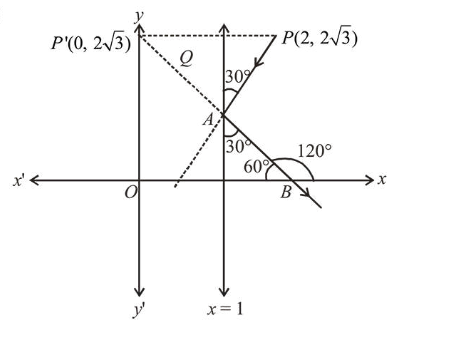# A ray of light coming from the pointQuestion:

A ray of light coming from the point $(2,2 \sqrt{3})$ is incident at an angle $30^{\circ}$ on the line $x=1$ at the point $\mathrm{A}$. The ray gets reflected on the line $x=1$ and meets $x$-axis at the point $\mathrm{B}$. Then, the line $\mathrm{AB}$ passes through the point:

1. (1) $\left(3,-\frac{1}{\sqrt{3}}\right)$

2. (2) $\left(4,-\frac{\sqrt{3}}{2}\right)$

3. (3) $(3,-\sqrt{3})$

4. (4) $(4,-\sqrt{3})$

Correct Option: , 3

Solution:Slope of $A B=\tan 120^{\circ}=-\sqrt{3}$

$\therefore$ Equation of line $A B$ (i.e. $B P^{\prime}$ ):

$y-2 \sqrt{3}=-\sqrt{3}(x-0)$

$\Rightarrow \sqrt{3} x+y=2 \sqrt{3}$

$\therefore$ Point $(3,-\sqrt{3})$ lies on line $A B$